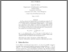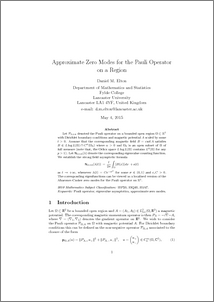Approximate zero modes for the Pauli operator on a region

Elton, Daniel (2016) Approximate zero modes for the Pauli operator on a region. Journal of Spectral Theory, 6 (2). pp. 373-413. ISSN 1664-039XPreview
PDF (approx2dzm_rev)
approx2dzm_rev.pdf - Accepted Version

Abstract

Let $\Pauli{\reg,t\magp}$ denoted the Pauli operator on a bounded open region $\reg\subset\R^2$ with Dirichlet boundary conditions and magnetic potential $A$ scaled by some $t>0$.Assume that the corresponding magnetic field $B=\mathrm{Curl}A$ satisfies $B\in L\log L(\Omega)\cap C^\alpha(\Omega_0)$ where $\alpha>0$ and $\Omega_0$ is an open subset of $\Omega$ of full measure (note that, the Orlicz space $L\log L(\Omega)$ contains $L^p(\Omega)$ for any $p>1$). Let $\mathsf{N}_{\Omega,tA}(\lambda)$ denote the corresponding eigenvalue counting function. We establish the strong field asymptotic formula$\mathsf{N}_{\Omega,tA}(\lambda(t))\,=\,\frac{t}{2\pi}\int_{\Omega}\lvert{B(x)}\rvert\,d x\;+o(t)$as $t\to+\infty$, whenever $\lambda(t)=Ce^{-ct^\sigma}$ for some $\sigma\in(0,1)$ and $c,C>0$.The corresponding eigenfunctions can be viewed as a localised version of the Aharonov-Casher zero modes for the Pauli operator on $\mathbb{R}^2$.

Item Type:
Journal Article
Journal or Publication Title:
Journal of Spectral Theory
Uncontrolled Keywords:
/dk/atira/pure/subjectarea/aacsb/disciplinebasedresearch
Subjects:
Departments:
ID Code:
89224
Deposited By:
Deposited On:
15 Dec 2017 00:04
Refereed?:
Yes
Published?:
Published